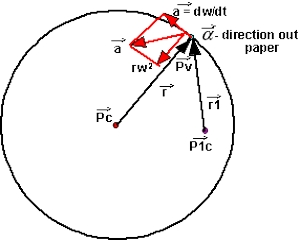# What is Angular Acceleration?

If the angular velocity of the body performing rotatory motion is non-uniform, then the body is said to possess angular acceleration.

The rate of change of angular velocity is called angular acceleration.

If the angular velocity of a body moving in a circular path changes from ω1 to ω2 in time t then its angular acceleration is

α = dω/dt = d/dt(dθ/dt) = d2θ/dt2 = (ω2 – ω1)/tThe angular acceleration is measured in terms of rad s-2 and its dimensional formula is T-2.